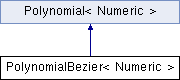clipper
PolynomialBezier< Numeric > Class Template Reference

Represents a polynomial in the Bernstein-Bezier basis using Bezier coefficients. More...

Inheritance diagram for PolynomialBezier< Numeric >:List of all members.

Public Member Functions

PolynomialBezier (const std::vector< Numeric > &coefficient)
unsigned int degree () const
Return degree of the polynomial.
Numeric coefficient (unsigned int i) const
Return a Bezier coefficient.
std::string toString (const std::string &T="") const
Return polynomial as latex math string.
std::string toPolygon () const
Return Bezier coefficients as a polygon.
std::vector< std::pair
< Numeric, Numeric > >
getConvexHull () const
Return the convex hull of the Bezier coefficients calculated using Jarvis's march.
std::vector< Numeric > getConvexHullIntersection () const
Return the convex hull of the Bezier coefficients calculated using Jarvis's march.
std::string toConvexHullString () const
Return the convex hull of the Bezier coefficients for plotting.
class PolynomialStandard< Numeric > toStandard () const
Transform the polynomial from Bernstein-Bezier basis back to the standard monomial basis.
Numeric evaluate (Numeric X) const
Calculate the polynomial's value at the position X using the schema of de Casteljau.
std::pair< PolynomialBezier,
PolynomialBezier
deCasteljauSplit (Numeric t0) const
Similar to evaluate: run de Casteljau's algorithm and output the Bezier coefficients of interval [0,t0] and [t0,1] as separate polynomial objects.
std::vector< Numeric > findRoots () const
Calculate all real roots for a quadratic or cubic polynomial in Bezier representation (according to Remark 3 in ).
PolynomialBezier interpolateDegree (int k) const
This function is the heart of the root finding algorithm: reduce or raise the degree of this polynomial to k.
PolynomialBezier interpolateDegree (const class Matrix< Numeric > &m) const
This function is the heart of the root finding algorithm: reduce or raise the degree of this polynomial by applying a matrix.
Numeric maxCoefficientDeltaTo (const PolynomialBezier &b) const
Compare two Bezier polynomials of same degree and return the maximum difference between pairs of coefficients.
PolynomialBezier operator+ (const Numeric &v) const
Create a new polynomial shifted upwards by adding v to all coefficients.
PolynomialBezier operator- (const Numeric &v) const
Create a new polynomial shifted downwards by subtracting v from all coefficients.

Private Attributes

std::vector< Numeric > m_coefficient
The n+1 Bezier coefficients to the Bernstein polynomials basis ()

Detailed Description

template<typename Numeric> class PolynomialBezier< Numeric >

Represents a polynomial in the Bernstein-Bezier basis using Bezier coefficients.

Definition at line 1061 of file clipper.cc.

Constructor & Destructor Documentation

template<typename Numeric>
 PolynomialBezier< Numeric >::PolynomialBezier ( const std::vector< Numeric > & coefficient ) [inline, explicit]

Definition at line 1072 of file clipper.cc.

Member Function Documentation

template<typename Numeric>
 Numeric PolynomialBezier< Numeric >::coefficient ( unsigned int i ) const [inline]

Return a Bezier coefficient.

Definition at line 1084 of file clipper.cc.

template<typename Numeric>
 std::pair PolynomialBezier< Numeric >::deCasteljauSplit ( Numeric t0 ) const [inline]

Similar to evaluate: run de Casteljau's algorithm and output the Bezier coefficients of interval [0,t0] and [t0,1] as separate polynomial objects.

This is later used to branch when finding roots.

Definition at line 1300 of file clipper.cc.

template<typename Numeric>
 unsigned int PolynomialBezier< Numeric >::degree ( ) const [inline]

Return degree of the polynomial.

Definition at line 1078 of file clipper.cc.

template<typename Numeric>
 Numeric PolynomialBezier< Numeric >::evaluate ( Numeric X ) const [inline]

Calculate the polynomial's value at the position X using the schema of de Casteljau.

Definition at line 1277 of file clipper.cc.

template<typename Numeric>
 std::vector PolynomialBezier< Numeric >::findRoots ( ) const [inline]

Calculate all real roots for a quadratic or cubic polynomial in Bezier representation (according to Remark 3 in ).

Imaginary roots are ignored.

Instead of using the Cardano's formulas in Bezier form as in , we return to the monomial base case for calculating the roots

Definition at line 1331 of file clipper.cc.

template<typename Numeric>
 std::vector< std::pair > PolynomialBezier< Numeric >::getConvexHull ( ) const [inline]

Return the convex hull of the Bezier coefficients calculated using Jarvis's march.

Definition at line 1126 of file clipper.cc.

template<typename Numeric>
 std::vector PolynomialBezier< Numeric >::getConvexHullIntersection ( ) const [inline]

Return the convex hull of the Bezier coefficients calculated using Jarvis's march.

Definition at line 1178 of file clipper.cc.

template<typename Numeric >
 PolynomialBezier< Numeric > PolynomialBezier< Numeric >::interpolateDegree ( int k ) const

This function is the heart of the root finding algorithm: reduce or raise the degree of this polynomial to k.

Apply a degree rasing of reducing matrix, where the matrix has to be constructed before application.

Definition at line 1651 of file clipper.cc.

template<typename Numeric>
 PolynomialBezier PolynomialBezier< Numeric >::interpolateDegree ( const class Matrix< Numeric > & m ) const

This function is the heart of the root finding algorithm: reduce or raise the degree of this polynomial by applying a matrix.

template<typename Numeric>
 Numeric PolynomialBezier< Numeric >::maxCoefficientDeltaTo ( const PolynomialBezier< Numeric > & b ) const [inline]

Compare two Bezier polynomials of same degree and return the maximum difference between pairs of coefficients.

Definition at line 1395 of file clipper.cc.

template<typename Numeric>
 PolynomialBezier PolynomialBezier< Numeric >::operator+ ( const Numeric & v ) const [inline]

Create a new polynomial shifted upwards by adding v to all coefficients.

Definition at line 1406 of file clipper.cc.

template<typename Numeric>
 PolynomialBezier PolynomialBezier< Numeric >::operator- ( const Numeric & v ) const [inline]

Create a new polynomial shifted downwards by subtracting v from all coefficients.

Definition at line 1416 of file clipper.cc.

template<typename Numeric>
 std::string PolynomialBezier< Numeric >::toConvexHullString ( ) const [inline]

Return the convex hull of the Bezier coefficients for plotting.

Definition at line 1258 of file clipper.cc.

template<typename Numeric>
 std::string PolynomialBezier< Numeric >::toPolygon ( ) const [inline]

Return Bezier coefficients as a polygon.

Definition at line 1112 of file clipper.cc.

template<typename Numeric >
 PolynomialStandard< Numeric > PolynomialBezier< Numeric >::toStandard ( ) const

Transform the polynomial from Bernstein-Bezier basis back to the standard monomial basis.

Definition at line 1451 of file clipper.cc.

template<typename Numeric>
 std::string PolynomialBezier< Numeric >::toString ( const std::string & T = "" ) const [inline]

Return polynomial as latex math string.

Definition at line 1091 of file clipper.cc.

Member Data Documentation

template<typename Numeric>
 std::vector PolynomialBezier< Numeric >::m_coefficient [private]

The n+1 Bezier coefficients to the Bernstein polynomials basis ()

Definition at line 1067 of file clipper.cc.

The documentation for this class was generated from the following file: